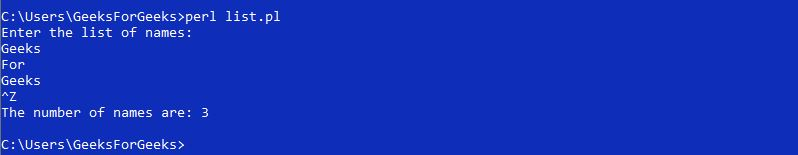# Perl | List Context Sensitivity

Introduction
In Perl, function calls, terms, and statements have inconsistent explications which rely upon its Context. There are two crucial Contexts in Perl, namely List Context and Scalar Context. In a list context, Perl gives the list of elements. But in a scalar context, it returns the number of elements in the array.
Perl presumes List values in “List Context”, where as the list could have any number of elements or it can have only one element or it can even be desolated.

Creating List Context

List Context can be generated with the use of Arrays and Lists.

• Assignment to an array:
Example:

`@y = LIST;`
`@y = @z;`
`@y = localtime();`

Here, localtime() is a function name in Perl, which unveils number depiction of time in array.

• Assignment to a list:
Example:

`(\$x, \$y) = LIST;`
`(\$x) =  LIST;`

Here, List can moreover create List Context even if the List has only one element.

Example:

 `#!/usr/bin/perl ` `# Perl program of creating List Context ` ` `  `# array of elements ` `my` `@CS` `= (``'geeks'``, ``'for'``, ``'geeks'``, ``'articles'``);  ` `             `  `# Assignment to a list  ` `my` `(``\$x``, ``\$y``) = ``@CS``;  ` ` `  `# Assignment to an Array ` `my` `@z` `= ``@CS``;         ` `     `  `# Assignment of a function ` `# to an Array ` `my` `@t` `= ``localtime``(); ` `             `  `# Displays two elements of an ` `# array in List ` `print` `"\$x \$y\n"``; ` ` `  `# Displays an array of elements ` `print` `"@z\n"``;     ` ` `  `# Displays time stored in array  ` `# in number format ` `print` `@t``; `

Output:

```geeks for
geeks for geeks articles
201761121191690
```

Here, in assignment to List there are two scalars in the List i.e, \$x and \$y, so only two elements of the array are assigned.

Arrays in List Context:

In order to provoke List Context using an array, we need to assign an array to another array.
Example:

 `#!/usr/bin/perl ` ` `  `# Program for arrays in List Context ` `use` `strict; ` `use` `warnings; ` `use` `5.010; ` ` `  `my` `@x` `= (``'computer_'``, ``'science_'``, ``'portal_'``, ` `         ``'for_'``, ``'GeeksforGeeks'``); ` ` `  `# Assignment of an array to  ` `# another array ` `my` `@y` `= ``@x``;  ` ` `  `# Printing the new array ` `print` `@y``; `

Output:

```computer_science_portal_for_GeeksforGeeks
```

Here, elements from one array are copied to another array.

Use of if-statement in List Context

if-statement is used in a List context to display the statements enclosed within ‘if’ only when there are elements present in the array.
Example:

 `#!/usr/bin/perl ` ` `  `# Program to display content of if-statement ` `use` `strict; ` `use` `warnings; ` `use` `5.010; ` ` `  `my` `@x` `= (``'G'``, ``'f'``, ``'G'``); ` `  `  `# Statement within 'if' will be executed  ` `# only if the array is not empty ` `if` `(``@x``) ` `{ ` `    ``print` `"GeeksforGeeks"``; ` `} `

Output:

```GeeksforGeeks
```

Here, if the stated array has some number of elements then if-condition is true and it provokes the content of the if-statement but if the array is empty then the if-condition is false so it does not execute the statements within if-statement.

“STDIN” is a readline operator in Perl. In order to place readline operator in List Context it is required to designate this operator to an array.
Example:

 `#!/usr/bin/perl ` `use` `strict; ` `use` `5.010; ` ` `  `# Asking user to provide input  ` `print` `"Enter the list of names:\n"``; ` ` `  `# Getting input from user  ` `my` `@y` `= ; ` ` `  `# Used to remove extra line of spaces ` `chomp` `@y``; ` ` `  `# Printing the required output ` `print` `"The number of names are: "` `.  ` `                 ``scalar``(``@y``) . ``"\n"``;  `

Output:Here is how this program works:
Step 1: Enter the names which are to be stored in the array one by one with the use of enter key.
Step 2: Press Ctrl-D in Linux systems whereas Ctrl-Z in Windows system to indicate the ending of input.
Step 3: chomp is used to remove the extra line added after each input.
Step 4: Printing the number of elements in the array with the use of scalar because “an array in Scalar Context” can only return the length of an array.

My Personal Notes arrow_drop_upCheck out this Author's contributed articles.

If you like GeeksforGeeks and would like to contribute, you can also write an article using contribute.geeksforgeeks.org or mail your article to contribute@geeksforgeeks.org. See your article appearing on the GeeksforGeeks main page and help other Geeks.

Please Improve this article if you find anything incorrect by clicking on the "Improve Article" button below.

Article Tags :
Practice Tags :

Be the First to upvote.

Please write to us at contribute@geeksforgeeks.org to report any issue with the above content.